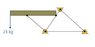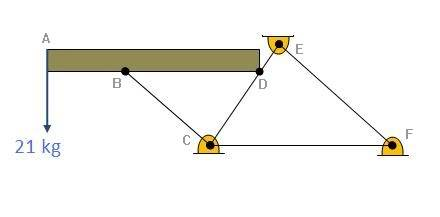# Another simple truss problem

## Homework Statement

Hi guys,
Below is a picture of what I am given. I need to calculate the support reaction forces and the stress in each truss. Having problems resolving the offset weight.

## Homework Equations

All the supports are pin supports. Any ideas how to get started?

## The Attempt at a Solution

Can't even get started as i have too many variables compared with equations to initially find the Rx Ry support forces.

#### Attachments

•statics.jpg
5.2 KB · Views: 380
Anyone?

Is the noly way to do it is to remove one of the supports?

I hope you realize that we don't get to see the picture until it is approved. It usually takes a good part of the day.
If you are in a big rush, you could upload the picture to an image server and post the link.

How long does it take to get approved?

...
...It usually takes a good part of the day.

Hey guys,
Just bumping my question as I hope the pic is up now.
Any suggestions would be helpfull, will try to answer any others too.

The picture is now approved.

It does not, however, show the elastic properties, E and A, nor the geometric dimensions required to do the calculations. I assume the horizontal beam is infinitely rigid.

To be able to make discussions, I have named the joints A, B, C, D, E, F, starting from left to right, and where the load 21 Kg is applied at A.Could you please supply the member properties (E and A) as well as details of the geometry?

From the diagram, it does not look like it is a linear problem, because the point D can have a transversal displacement, which has a non-linear relationship with the axial forces of CD and DE.

For the solution, you can proceed as follows:
Unknowns: 4 displacement, Bx, By, Dx, Dy
Equations:
Equilibrium of forces at points B and D
Axial force on member BC
Moments of forces and reactions.

If BD is supposed to be rigid, a large value of A and/or E must be applied to member BD.

Hope this will give you a kickstart.

Could you also tell me if your current course is on indeterminate structures?

AD is just to show that the force due to weight is not directly on B. It's not rigid or attatched to member BD (sorry it looks like it in the picture).

CF=1m
EF=1.5m
CE=1.4m
CB=1.6m

It's supposed to be a truss problem, just finding the reaction forces in supports and internal truss forces. Not sure about deflection.
E is 20GPa and A is 111mm2.

Looking for method of joints + method of sections?
I'm getting a trivial result with a matrix inverse unidentified solution of 6 equations and 6 unkowns. zzzz.

Is E on a horizontal slider? If it is, then the structure is unstable.
If it is not, the joint F and members CF and EF are not stressed, i.e. the given load has no effect on them.
Also, for the structure to be stable, the rigid beam AD is required. Otherwise point B will spin counter-clockwise around C.

If we disregard non-linear effects, and the members CF and EF, then you are left with the structure ABCDE where C and E are pinned.

If CDE is rigid, then the reactions at C and E are vertical.
You can calculate these vertical reactions by taking moments about E and C respectively.